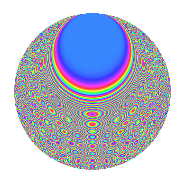# Properties

 Label 1350.2.wLevel 1350 Weight 2 Character orbit w Rep. character $$\chi_{1350}(53,\cdot)$$ Character field $$\Q(\zeta_{20})$$ Dimension 320 Sturm bound 540

# Related objects

## Defining parameters

 Level: $$N$$ = $$1350 = 2 \cdot 3^{3} \cdot 5^{2}$$ Weight: $$k$$ = $$2$$ Character orbit: $$[\chi]$$ = 1350.w (of order $$20$$ and degree $$8$$) Character conductor: $$\operatorname{cond}(\chi)$$ = $$75$$ Character field: $$\Q(\zeta_{20})$$ Sturm bound: $$540$$

## Dimensions

The following table gives the dimensions of various subspaces of $$M_{2}(1350, [\chi])$$.

Total New Old
Modular forms 2256 320 1936
Cusp forms 2064 320 1744
Eisenstein series 192 0 192

## Trace form

 $$320q + 4q^{7} + O(q^{10})$$ $$320q + 4q^{7} - 4q^{10} - 24q^{13} + 80q^{16} - 40q^{19} - 36q^{22} - 8q^{25} - 16q^{28} + 80q^{34} - 24q^{37} + 16q^{40} + 72q^{43} + 24q^{52} + 12q^{55} - 16q^{58} + 72q^{67} + 76q^{70} + 28q^{73} + 80q^{79} + 48q^{82} + 176q^{85} + 4q^{88} - 8q^{97} + O(q^{100})$$

## Decomposition of $$S_{2}^{\mathrm{new}}(1350, [\chi])$$ into newform subspaces

The newforms in this space have not yet been added to the LMFDB.

## Decomposition of $$S_{2}^{\mathrm{old}}(1350, [\chi])$$ into lower level spaces

$$S_{2}^{\mathrm{old}}(1350, [\chi]) \cong$$ $$S_{2}^{\mathrm{new}}(75, [\chi])$$$$^{\oplus 6}$$$$\oplus$$$$S_{2}^{\mathrm{new}}(150, [\chi])$$$$^{\oplus 3}$$$$\oplus$$$$S_{2}^{\mathrm{new}}(225, [\chi])$$$$^{\oplus 4}$$$$\oplus$$$$S_{2}^{\mathrm{new}}(450, [\chi])$$$$^{\oplus 2}$$$$\oplus$$$$S_{2}^{\mathrm{new}}(675, [\chi])$$$$^{\oplus 2}$$

## Hecke Characteristic Polynomials

There are no characteristic polynomials of Hecke operators in the database